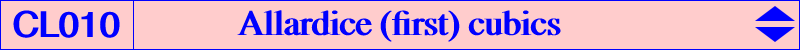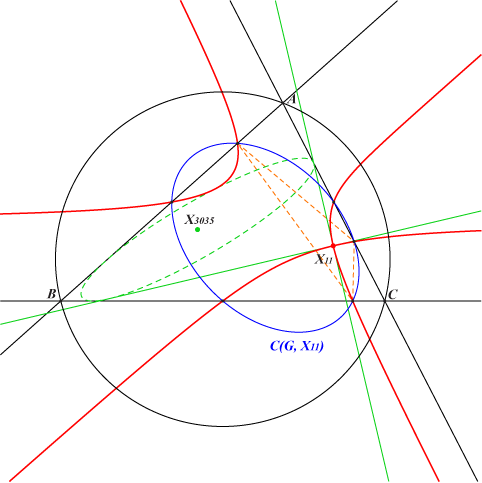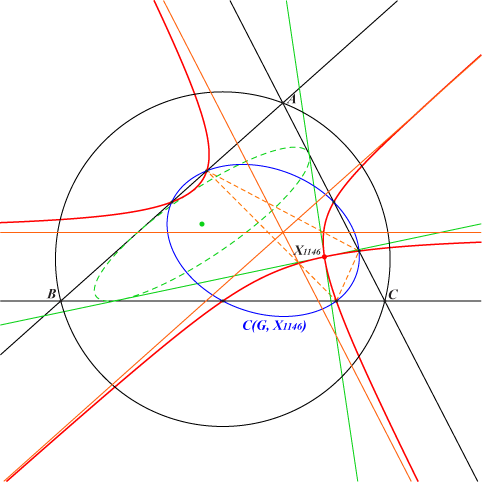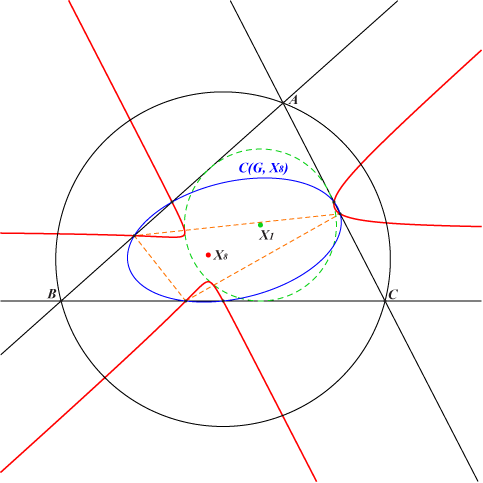Robert Allardice : On a cubic curve connected with the triangle, Proceedings of the Edinburgh Mathematical Society, Volume 19, pp 62 - 65 (1900). *** We know that the (real or not) asymptotes of a circum-conic meet each sideline of ABC at two points symmetric with respect to the midpoint of the side. Given a fixed finite point P = (u : v :w), the Allardice cubic A1(P) is the locus of the center of a circum-conic such that one of its asymptotes passes through P. The other asymptote envelopes the in-conic with center the complement of P and perspector the isotomic conjugate of P. The tangents at P to A1(P) are those drawn from P to this in-conic. They are perpendicular if and only if P lies on the nine point circle. Note that this in-conic is tritangent to A1(P), the points of tangency being all real when P is an acnode on the cubic. A1(P) contains the following points : P which is singular the midpoints of ABC the points at infinity of the sidelines of ABC the vertices of the cevian triangle of P. A1(P) is a K+ if and only if P lies on the inscribed Steiner ellipse. The general equation of A1(P) is : ∑vwx(y^2+z^2-x^2) = (u^2+v^2+w^2)xyz or equivalently : (x+y+z) ∑vwx(y+z-x) = (u+v+w)^2 xyz, in which we recognize the equation of the bicevian conic C(G,P). K051 = A1(H) is an example of such cubic but the most remarkable is probably K203 = A1(X115) since X(115) lies simultaneously on the inscribed Steiner ellipse and on the nine point circle. Another remarkable example is K219 = A1(G).   The following figures show examples of the special cases discussed above.With P = X(11), the Feuerbach point on the nine-point circle, the nodal tangents are perpendicular and tangent to the in-conic with center X(3035).With P = X(1146) on the Steiner inellipse, the cubic is a K+ with three concurring asymptotes. When P traverses the Steiner inellipse, the common point of the asymptotes lies on a quartic with three cusps namely the midpoints of ABC.With P = X(8), the Nagel point, the nodal tangents are tangent to the incircle but not necessarily real as shown on the figure. In this case, the cubic is tritangent to the incircle with three real contact points.If we replace "asymptote" by "axis" in the definition of A1(P) we obtain another cubic which is actually the complement of the Lemoine cubic K(aP) where aP denotes the anticomplement of P. See the FG paper Lemoine generalized cubics. See CL047 for similar cubics related to inconics.